## 蓝桥杯重点（C/C++）（随时更新）

1  技巧

1.1  取消同步（节约时间，甚至能多骗点分，最好每个程序都写上）

1.2  万能库（可能会耽误编译时间，但是省脑子）

1.3  蓝桥杯return 0千万别忘了写！！

1.4  编译设置（Dev C++）

1.5  memset填充函数

1.6  时间复杂度

1.6.1  常数阶  O（1）

1.6.2  对数阶  O（logn）

1.6.3  线性阶  O（n）

1.6.4  线性对数阶  O(nlogn)

1.6.5  多重循环  O（n^k）

1.7  剪枝

1.8  find函数

2  基本算法及技巧

2.1  BFS（宽度优先搜索）

2.2  DFS（深度优先搜索）

2.3  最大公约数和最小公倍数

2.4  进制转换

2.4.1  十进制为媒介（常用型）

2.4.2  二进制为媒介（技巧型）

2.5  二进制表示法

2.6  背包问题

2.6.1  01背包问题

2.6.2  多重背包问题（每种物品有多件）

2.6.3  完全背包问题（每种物品有无数件）

2.7  动态规划（DP）

2.8  贪心

2.9  分治（以后更新）

2.10  数字分拆到数组中

2.11  数字和字符串的互化

2.12  排序

3  STL

3.1  队列（queue）

3.2  链表（list）

3.3  优先队列（priority queue）

3.4  向量/动态数组（vector）

3.5  栈（stack）

3.6  集合（set） （要求没有重复元素）

3.7  集合/映射/键值对（map）

3.8  迭代器

# 1  技巧

## 1.1  取消同步（节约时间，甚至能多骗点分，最好每个程序都写上）

``````ios::sync_with_stdio(false);
cin.tie(0);
cout.tie(0);``````

## 1.2  万能库（可能会耽误编译时间，但是省脑子）

``#include <bits/stdc++.h>``

## 1.4  编译设置（Dev C++）

（1）工具->编译选项->编译器->编译时加入以下命令->调成C99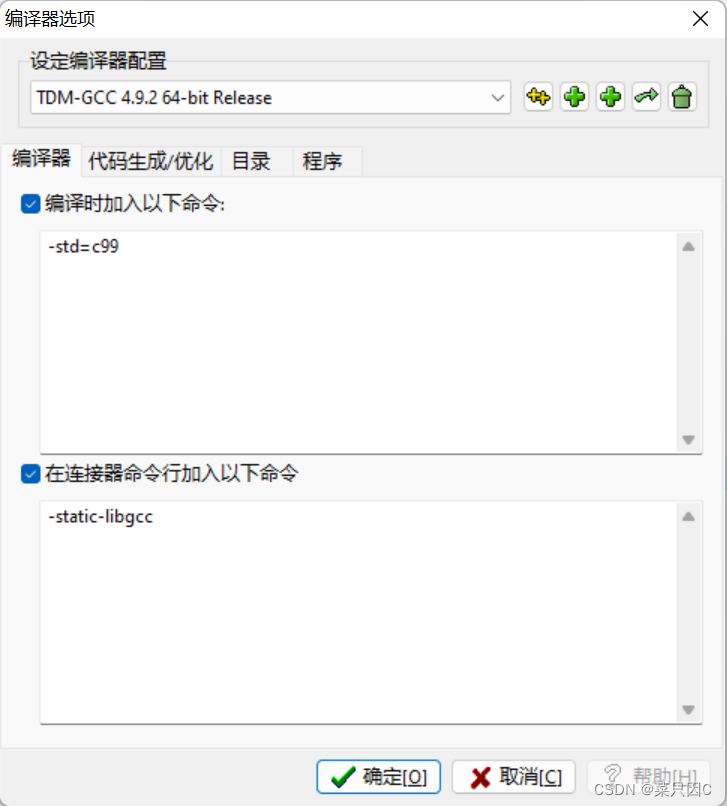（2）工具->编译选项->代码生成/优化->代码生成->语言标准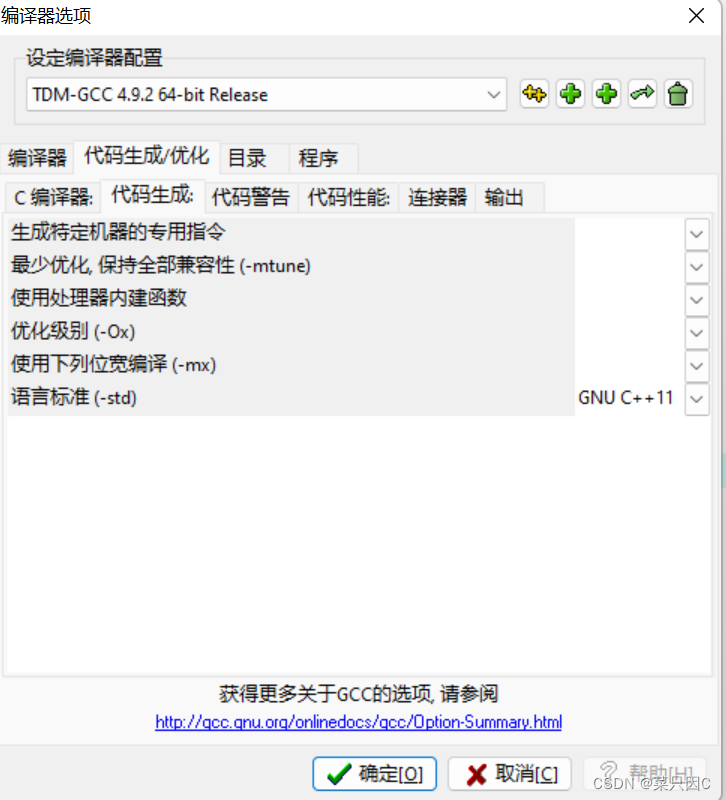## 1.5  memset填充函数

``````#include <bits/stdc++.h>
using namespace std;
int a;
int main()
{
memset(a,-1,sizeof(a));
for(int i=0;i<10;i++)
{
cout<<a[i]<<endl;
}
return 0;
}``````

## 1.6  时间复杂度

(1条消息) K倍区间（蓝桥杯2017年第八届省赛B组第十题）（C/C++）_菜只因C的博客-CSDN博客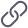https://blog.csdn.net/m0_71934846/article/details/128434135?spm=1001.2014.3001.5501

### 1.6.1  常数阶  O（1）

``````int i=1;
int j=2;
int m=i+j;``````

### 1.6.2  对数阶  O（logn）

``````int i=1;
while(i<n)
{
i=i*2;
}``````

### 1.6.3  线性阶  O（n）

``````for(int i=0;i<n;i++)
{
cout<<i<<endl;
)``````

### 1.6.4  线性对数阶  O(nlogn)

``````for(int m=1;m<n;m++)
{
int i=1;
while(i<n)
{
i=i*2;
}
}``````

k为循环层数

## 1.8  find函数

``````#include <bits/stdc++.h>
using namespace std;

int main()
{
int a={2,6,8,1,3,7,5,1,0,11};
cout<<find(a+0,a+5,8)<<endl;//打印类似0x地址
cout<<find(a+0,a+5,8)-a<<endl;//打印数组【】内的序号
return 0;
}``````

PI=atan（1.0）*4

# 2  基本算法及技巧

## 2.1  BFS（宽度优先搜索）

(1条消息) 全球变暖（蓝桥杯2018年省赛B组试题I）（C/C++）_菜只因C的博客-CSDN博客https://blog.csdn.net/m0_71934846/article/details/128434254?spm=1001.2014.3001.5502

## 2.2  DFS（深度优先搜索）

http://t.csdn.cn/ANnS1

（1）确定 边界    if（）return；

（2）进入for循环

（3）判断是否搜索过  if（vis[]）vis[]=true; dfs();  vis[]=false;

(3条消息) 凑算式（蓝桥杯2016年省赛B组第三题）（C/C++）_菜只因C的博客-CSDN博客https://blog.csdn.net/m0_71934846/article/details/128434004?spm=1001.2014.3001.5502

## 2.3  最大公约数和最小公倍数

``````#include <bits/stdc++.h>
using namespace std;

int main()
{
cout<<__gcd(25,5);
return 0;
}``````

``````#include <bits/stdc++.h>
using namespace std;

int lcm(int a,int b)
{
return a*b/__gcd(a,b);
}
int main()
{
cout<<lcm(25,5);
return 0;
}``````

## 2.4  进制转换

### 2.4.1  十进制为媒介（常用型）

(8条消息) 任意进制转换成十进制/十进制转换成任意进制（ASCII码法）（C/C++）_菜只因C的博客-CSDN博客https://blog.csdn.net/m0_71934846/article/details/128297645?spm=1001.2014.3001.5502

### 2.4.2  二进制为媒介（技巧型）

(2条消息) 十六进制转八进制（蓝桥杯基础练习C/C++）_菜只因C的博客-CSDN博客https://blog.csdn.net/m0_71934846/article/details/128745875?csdn_share_tail=%7B%22type%22%3A%22blog%22%2C%22rType%22%3A%22article%22%2C%22rId%22%3A%22128745875%22%2C%22source%22%3A%22m0_71934846%22%7D

## 2.5  二进制表示法

(8条消息) 蓝桥杯试题 算法训练 无聊的逗（C/C++）_菜只因C的博客-CSDN博客https://blog.csdn.net/m0_71934846/article/details/128717938?spm=1001.2014.3001.5502

## 2.6  背包问题

### 2.6.1  01背包问题

``````#include<bits/stdc++.h>

using namespace std;

int v;    // 体积
int w;    // 价值
int f;  // f[i][j], j体积下前i个物品的最大价值

int main()
{
int n, m;
cin >> n >> m;
for(int i = 1; i <= n; i++)
cin >> v[i] >> w[i];

for(int i = 1; i <= n; i++)
for(int j = 1; j <= m; j++)
{
//  当前背包容量装不进第i个物品，则价值等于前i-1个物品
if(j < v[i])
f[i][j] = f[i - 1][j];
// 能装，需进行决策是否选择第i个物品
else
f[i][j] = max(f[i - 1][j], f[i - 1][j - v[i]] + w[i]);
}

cout << f[n][m] << endl;

return 0;
}

``````

### 2.6.3  完全背包问题（每种物品有无数件）

``````#include<iostream>
using namespace std;
const int N = 1010;
int f[N];
int v[N],w[N];
int main()
{
int n,m;
cin>>n>>m;
for(int i = 1 ; i <= n ;i ++)
{
cin>>v[i]>>w[i];
}

for(int i = 1 ; i<=n ;i++)
for(int j = v[i] ; j<=m ;j++)
{
f[j] = max(f[j],f[j-v[i]]+w[i]);
}
cout<<f[m]<<endl;
}``````

## 2.7  动态规划（DP）

(1条消息) 拿金币（蓝桥杯算法训练）（C/C++)_菜只因C的博客-CSDN博客https://blog.csdn.net/m0_71934846/article/details/128456365?spm=1001.2014.3001.5502

## 2.10  数字分拆到数组中

(2条消息) 把一个数字分拆存到数组内（C/C++）_菜只因C的博客-CSDN博客https://blog.csdn.net/m0_71934846/article/details/128698111?spm=1001.2014.3001.5501

## 2.11  数字和字符串的互化

(2条消息) 超级质数（蓝桥杯C/C++算法赛）_菜只因C的博客-CSDN博客https://blog.csdn.net/m0_71934846/article/details/128723978?csdn_share_tail=%7B%22type%22%3A%22blog%22%2C%22rType%22%3A%22article%22%2C%22rId%22%3A%22128723978%22%2C%22source%22%3A%22m0_71934846%22%7D

## 2.12  排序

(4条消息) 找字符串中最大字符（三种快速方法）_求字符串中最大的字符_菜只因C的博客-CSDN博客https://blog.csdn.net/m0_71934846/article/details/128457227?spm=1001.2014.3001.5501

# 3  STL

## 3.1  队列（queue）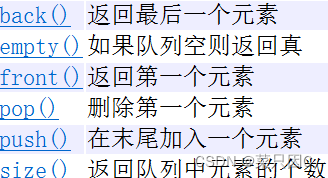## 3.2  链表（list）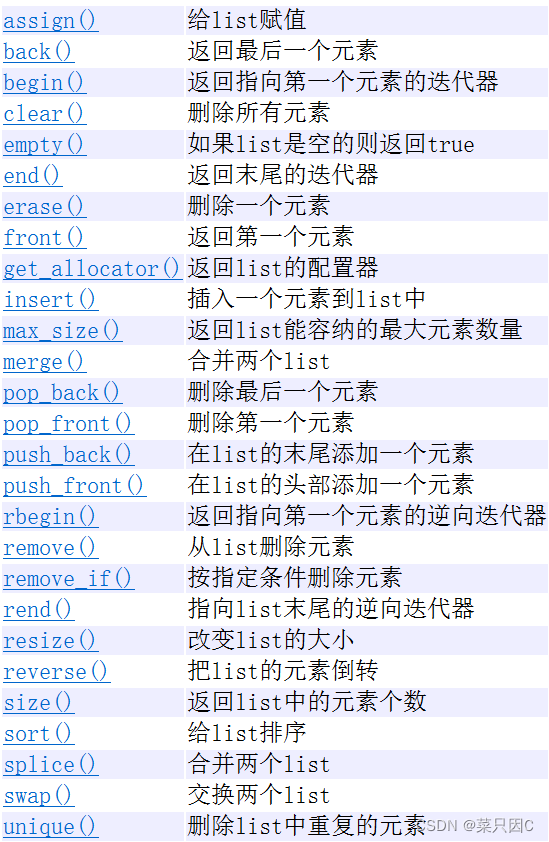## 3.3  优先队列（priority queue）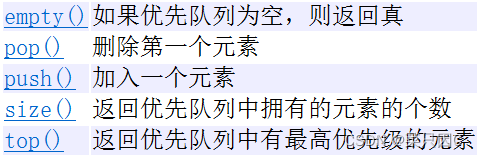优先队列默认大根堆（大到小排序），如果想从小到大排序，那么

<int,vector<int>,greater<int> >//升序排列（小根堆）

<int,vector<int>,less<int> >//降序排列（大根堆）

## 3.4  向量/动态数组（vector）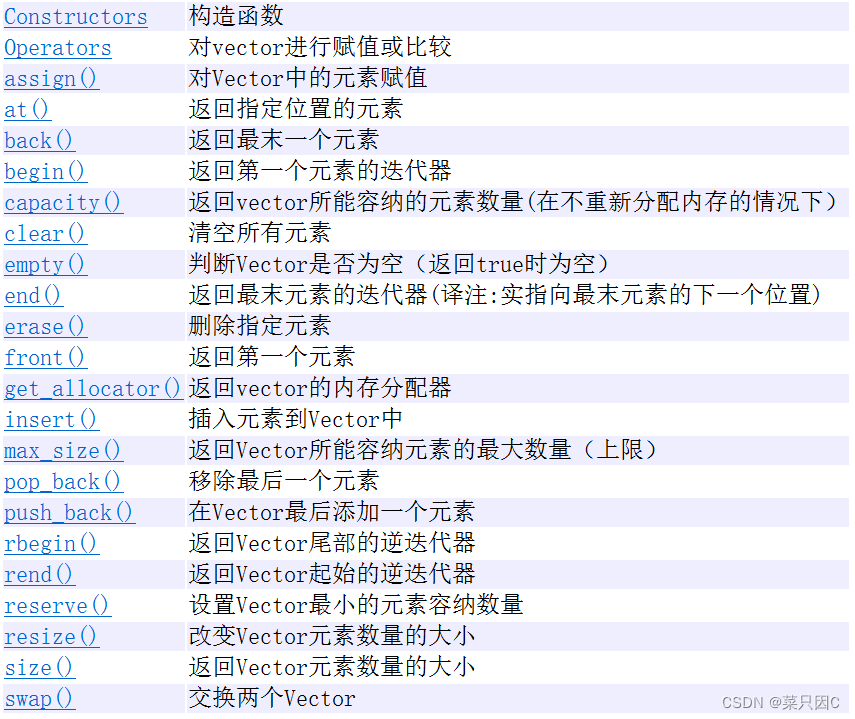## 3.5  栈（stack）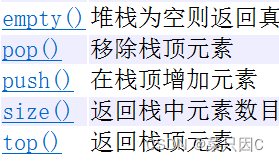## 3.6  集合（set） （要求没有重复元素）

set<int> s;//默认升序排列

set<int, greater<int>>  s2 = {3,2,5,1,4 ,3};//降序

set值不能修改（修改过后无法保证数据顺序）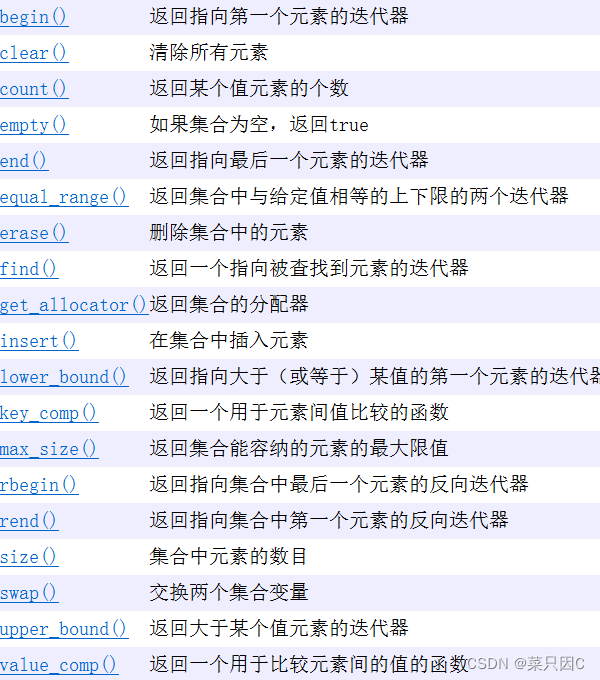## 3.7  集合/映射/键值对（map）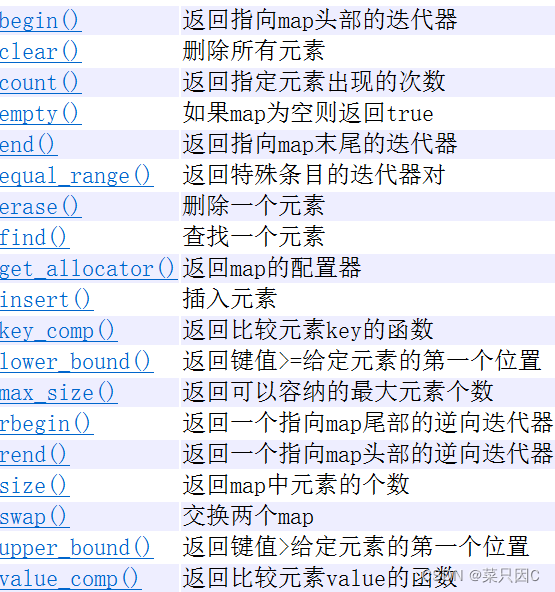## 3.8  迭代器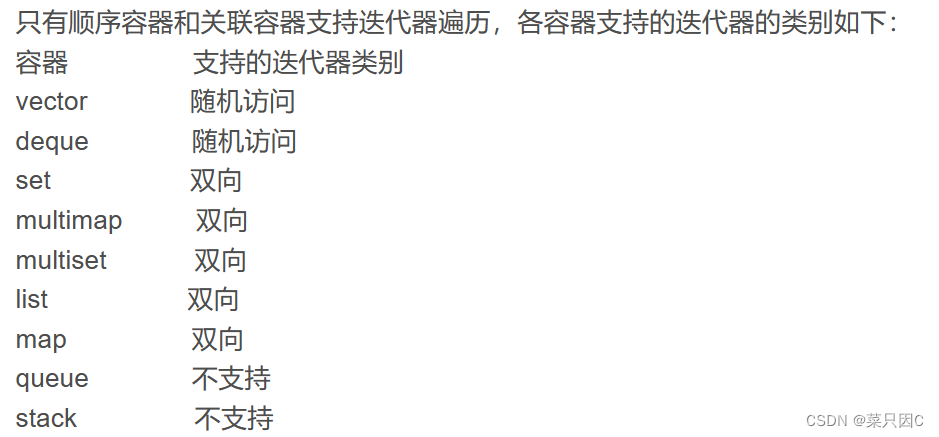模板：

``````#include<iostream>
#include<vector>
using namespace std;
int main()
{
vector<int> v;
v.push_back(11);
v.push_back(7);
vector<int>::iterator it = v.begin();

while(it!=v.end())
{
cout << *it <<"  ";
it++;
}
cout << endl;
return 0;
}

``````